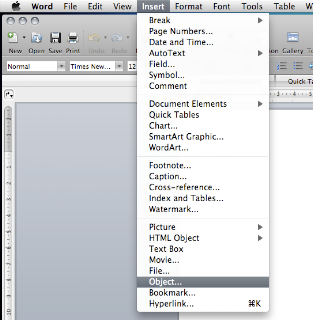# Create equations in Microsoft Word

Whether you are aware of it or not, Microsoft Word can not only handle words and images but also equations. The only catch here is that you need to have the equation editor installed. If not, you'll probably have to find the Microsoft Word CD lying around your house and install it. Once you do install it however it's quite easy to start creating equations for those gruesome physics papers.

The main component that allows Microsoft Word to insert equations is MathType, which is dumbed down a little bit, but is ample enough for most of the basic mathematical symbols. To insert equations into Microsoft Word all you need to do is this:

1. Open Microsoft Word
2. Click on Insert
3. Click Object4. Click on Microsoft Word Equation5. In the equation editor that opens up, you can then type in anything you want6. Simply the close the equation editor and the image in Microsoft Word will get updated to the mathematical formulas that you had entered.
7. Repeat.So there you have it. An easy way to do equations on Microsoft Word to make your labs look a lot more professional, and neat.

Got a question, tip or comment? Send them to beyondteck+question@gmail.com and we'll try to answer it in a blog post!